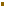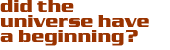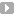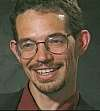### The No Boundary Proposal

The question of our most probable past formulated above is of course only answerable within the context of some definite theory which tells you the probability measure on past spacetimes. The usual arguments for eternal inflation are vague on this point, and basically just assume that for some reason a region existed in which there were the right conditions for inflation to start. Hartle and Hawking proposed an ansatz(which is a fancy way of saying they guessed!) the formula for relative probabilities. One can easily imagine an infinite number of possible ansatzes for the initial conditions of the Universe. At the present time many of these might be perfectly consistent with observation. But Hartle and Hawking’s proposal is appealing because it is based on general ideas which have a rationale beyond cosmology. In a strong sense I think it is ‘the most conservative thing you could do’. It may well fail precisely because it is too conservative. Space and time may be emergent rather than fundamental properties. Describing the Universe as a manifold may not be appropriate to its early moments. Nevertheless precisely because the no boundary proposal is not just cooked up to make inflation work, its failings and limitations may teach us something deeper about what is in fact required.

In physics we are now used to the fact that all we can ever predict in practice is probabilities. This was even true in classical physics, because nothing is ever measured perfectly and in consequence all predictions carry ‘error bars’. But in quantum physics the issue is more fundamental, because the quantities we use to describe the world, and which we need in order to predict its future or to extrapolate back into the past (like position and velocity of a particle) are in principle impossible to measure beyond a certain limiting accuracy. Only certain questions are allowed, and these involve correlations between physical quantities. Paradoxically, in spite of the limitations quantum mechanics imposes on our ability to predict classical properties of the world, quantum physics is actually a far more complete theory than classical physics because it predicts the correlations with perfect accuracy.

Hartle and Hawking proposed to use the formulation of quantum mechanics defined by Dirac and Feynman, called the path integral formulation, to define all cosmological correlators. This formulation is widely accepted and is the basis for modern treatments of gauge field theories, well tested in the laboratory. But it usually does not claim to solve the initial conditions problem. Usually the path integral formula is used to give the quantum mechanical amplitude to be in a state B at time tf, given that the system was in a state A at time ti.

There is one situation however, in which it becomes unnecessary to specify exactly what state the system was in. That is when the system is in thermal equilibrium. In this case, two beautiful things happen to the Dirac-Feynman formula. First, we identify the initial state A with the final state B, and sum over all possible states. Second, real time is continued to imaginary time, and the temperature of the system then replaces the role of the period of the system in imaginary time. In non-gravitational physics, one still needs to specify the temperature in order to specify all correlators. But in the context of gravity, the size of the system in imaginary time becomes a dynamical quantity to be determined by the theory itself. Thus Hartle and Hawking’s proposal can actually become a completely self-referential prescription for the initial conditions which does not need any input except the dynamical laws of physics (the Lagrangian).

It may seem paradoxical that quantum physics yields better defined probability measures than classical physics because in quantum physics certain quantities are inherently uncertain (Heisenberg’s ‘uncertainty principle’). But in fact the two aspects are closely related. For example, the state of a particle in a box is described in classical theory by its location and velocity, which can in principle be measured with absolute certainty. If we limit the energy of the particle, there are still an infinity of possible states for it to be in (for example differing infinitesimally in location). In quantum physics, however, if we limit the energy there are only a finite number of possible states. In all of these states both the location and velocity of the particle is to some extent uncertain.

The finiteness imposed by quantization is one aspect of obtaining a well defined probability measure. But there is another aspect, namely the box! If the box is infinite, there are an infinite number of possibilities for the particle and again the probability measure is ill defined. In many situations the precise nature of the box is irrelevant, as long as it is bigger than any relevant scale in the problem. But in some situations, it is all-important. For example, a gas particle in a box performs a random walk as it collides with other particles. For some questions, such as the average speed of the particle, the size of the box is irrelevant. But for others, such as ‘how far does it travel’, the walls of the box are all important. In cosmology, I want to argue that causality plays a role similar to the walls of the box.

Before proceeding let me list a few of the ‘technical’ difficulties to be faced in implementing Hartle and Hawking’s idea.

• Einstein gravity is non-renormalizable. This objection refers to the bad short distance properties of the theory. These are certainly important but not central to the discussion here. Theories such as supergravity with improved ultra-violet properties are not conceptually different as far as the problems we are discussing.

• The Euclidean Einstein action is not positive definite, and therefore the Euclidean path integral is ill defined. This is the ‘conformal factor’ problem in Euclidean quantum gravity and I shall return to it below. Recent work has shown that at least for some choices of physical variables, and to quadratic order, this problem is overcome.This means that at least to the extent that approximation calculations have so far been possible, the problem is overcome.

• The sum over topologies in four dimensions is likely to diverge, as happens in string theory. Most likely one will need some formulation in which manifolds of differing topologies are treated together.

These problems are formidable. The main hope, it seems to me, is that our Universe appears to be astonishingly simple, and well described by a classical solution of great symmetry with small fluctuations present at a level of a part in a hundred thousand. This suggests that we may be able to accurately describe it using perturbation theory and classical solutions.

Contributed by: Dr. Neil Turok

Cosmic QuestionsInflation and the Beginning of the Universe

The No Boundary ProposalIntroductionEvidence for InflationEternal InflationInstantonsEternal Inflation Isn't

#### Source:Neil Turok